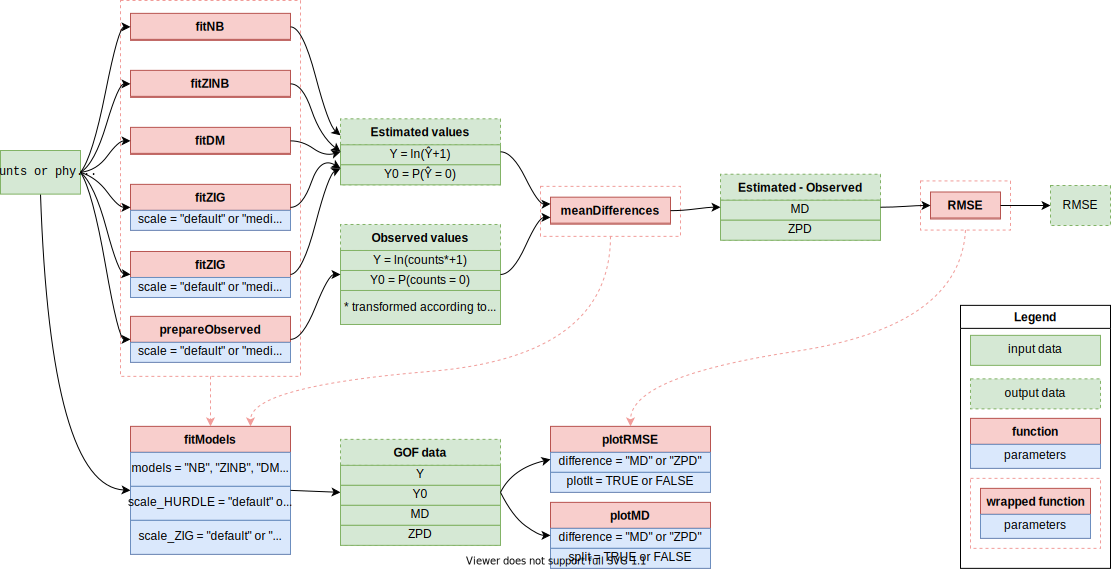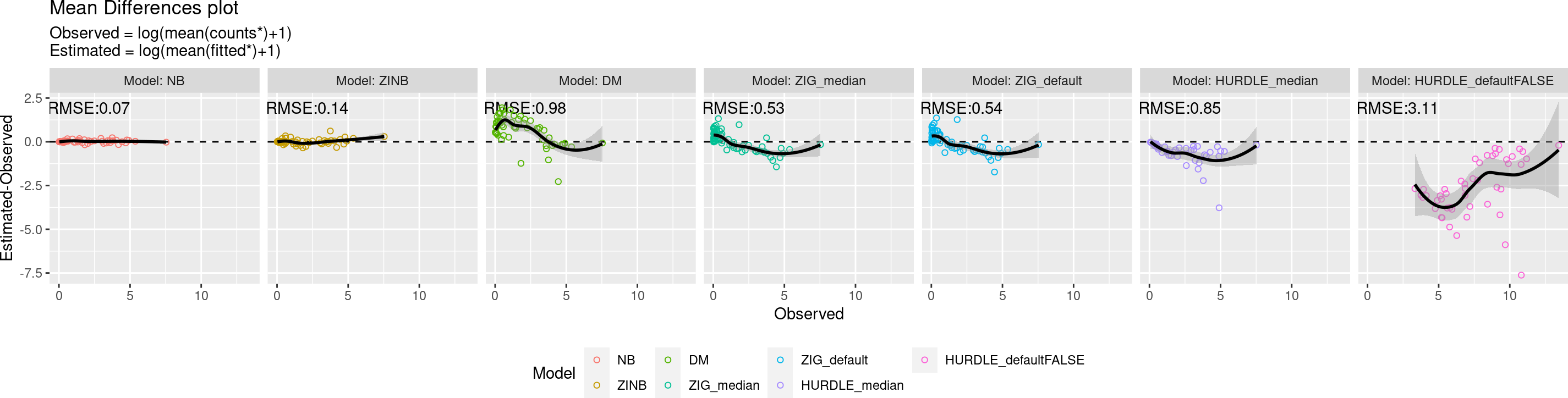# 1 Introduction

This vignette provides an introductory example on how to work with the analysis framework proposed in .

The package is named benchdamic, acronym for “BENCHmarking of Differential Abundance detection methods for MICrobial data”. Not only does the package structure allow the users to test a variety of commonly used methods for differential abundance analysis, but it also enables them to set benchmarks including custom methods on their datasets. Performances of each method are evaluated with respect to i) suitability of distributional assumptions, ii) ability to control false discoveries, iii) concordance of the findings, and iv) enrichment of differentially abundant microbial species in specific conditions. Each step of the assessment is flexible when it comes to the choice of differential abundance methods, their parameters, and input data types. Various graphic outputs lead the users to an informed decision when evaluating the most suitable method to use for their data.

# 2 Installation

The recommended way to install the benchdamic package is

devtools::install_github("mcalgaro93/benchdamic")

Then, let’s load some packages for basic functions and data.

library(benchdamic)
# Data management
library(phyloseq)
library(plyr)
# Graphics
library(ggplot2)
library(cowplot)

# 3 Goodness of Fit

We consider a homogeneous group of samples (e.g. only samples from a specific experimental condition, phenotype, treatment, body site…).

In this demonstrative example, datasets are downloaded from the HMP16SData Bioconductor package.

data("ps_stool_16S")
ps_stool_16S
## phyloseq-class experiment-level object
## otu_table()   OTU Table:         [ 71 taxa and 32 samples ]
## sample_data() Sample Data:       [ 32 samples by 7 sample variables ]
## tax_table()   Taxonomy Table:    [ 71 taxa by 6 taxonomic ranks ]
## phy_tree()    Phylogenetic Tree: [ 71 tips and 70 internal nodes ]
## 16S HMP data download
library(HMP16SData)
# Extracting V3-V5 16S sequenced regions' count data
ps_stool_16S_raw <- V35() %>%
subset(select = HMP_BODY_SUBSITE == "Stool" & # Only fecal samples
RUN_CENTER == "BI" & # Only sequenced at the BI RUN CENTER
SEX == "Male" & # Only male subject
VISITNO == 1 & # Only the first visit
!duplicated(RSID)) %>% # Duplicated SubjectID removal
as_phyloseq()
# Remove low depth samples
ps_stool_16S_pruned <- prune_samples(sample_sums(ps_stool_16S_raw) >= 10^3, ps_stool_16S_raw)
# Remove features with zero counts
ps_stool_16S_filtered <- filter_taxa(ps_stool_16S_pruned, function(x) {
sum(x > 0) > 0
}, 1)
# Collapse counts to the genus level
ps_stool_16S <- tax_glom(ps_stool_16S_filtered, taxrank = "GENUS")

## 3.2 GOF structure

As different methods rely on different statistical distributions to perform DA analysis, we assess the goodness of fit (GOF) of the statistical models underlying each method on a 16S dataset. For each model, we evaluate its ability to correctly estimate the average counts and the proportion of zeroes by taxon.

We consider five distributions: (1) the negative binomial (NB) used in edgeR and DeSeq2 [2,3], (2) the zero-inflated negative binomial (ZINB) used in ZINB-WaVE , (3) the truncated Gaussian Hurdle model of MAST , (4) the zero-inflated Gaussian (ZIG) mixture model of metagenomeSeq , and (5) the Dirichlet-Multinomial (DM) distribution underlying ALDEx2 .

The relationships between the functions used in this section are explained by the following diagram:Goodness of Fit - package structure

## 3.3 Model estimation

### 3.3.1 Negative Binomial and Zero-Inflated Negative Binomial Models

For any $$\mu \ge 0$$ and $$\theta > 0$$, let $$f_{NB}(\cdot;\mu,\theta)$$ denote the probability mass function (PMF) of the negative binomial (NB) distribution with mean $$\mu$$ and inverse dispersion parameter $$\theta$$, namely:$f_{NB} = \frac{\Gamma(y+\theta)}{\Gamma(y+1)\Gamma(\theta)}\left(\frac{\theta}{\theta+1} \right)^\theta\left(\frac{\mu}{\mu+\theta} \right)^y, \forall y \in \mathbb{N}$Note that another parametrization of the NB PMF is in terms of the dispersion parameter $$\psi = \theta^{-1}$$ (although $$\theta$$ is also sometimes called dispersion parameter in the literature). In both cases, the mean of the NB distribution is $$\mu$$ and its variance is:$\sigma^2 = \mu + \frac{\mu^2}{\theta} = \mu+\psi\mu^2$In particular, the NB distribution boils down to a Poisson distribution when $$\psi=0 \iff \theta=+ \infty$$.

For any $$\pi\in[0,1]$$, let $$f_{ZINB}(\cdot;\mu,\theta,\pi)$$ be the PMF of the ZINB distribution given by:

$f_{ZINB}(\cdot;\mu,\theta,\pi) = \pi\delta_0(y)+(1-\pi)f_{NB}(y;\mu,\theta), \forall y\in\mathbb{N}$

where $$\delta_0(\cdot)$$ is the Dirac function. Here, $$\pi$$ can be interpreted as the probability that a 0 is observed instead of the actual count, resulting in an inflation of zeros compared to the NB distribution, hence the name ZINB.

The packages we rely on to estimate these distributions on real count data are edgeR  and ZINB-WaVE  but we can easily call the benchdamic functions fitNB and fitZINB.

### 3.3.2 Zero-Inflated Gaussian Model

The raw count for sample j and feature i is denoted by $$c_{ij}$$. The zero-inflated model is defined for the continuity-corrected logarithm of the raw count data: $$y_{ij} = log_2(c_{ij}+1)$$ as a mixture of a point mass at zero $$I_{0}(y)$$ and a count distribution $$f_{count}(y;\mu,\sigma^2) \sim N(\mu,\sigma^2)$$. Given mixture parameters $$\pi_j$$, we have that the density of the ZIG distribution for feature i, in sample j with $$s_j$$ total counts is: $f_{ZIG}(y_{ij};s_j,\beta,\mu_i,\sigma^2_i) = \pi_j(s_j)\cdot I_{0}(y_{ij})+(1-\pi_j(s_j))\cdot f_{count}(y_{ij};\mu,\sigma^2)$

The mean model is specified as:$E(y_{ij})=\pi_{j} + (1-\pi_j)\cdot\left(b_{i0}+\eta_ilog_2\left( \frac{s_j^\hat{l}}{N}+1 \right) \right)$

In this case, parameter $$b_{i0}$$ is the intercept of the model while the term including the logged normalization factor $$log_2\left(\frac{s_j^\hat{l}}{N}+1 \right)$$ captures feature-specific normalization factors through parameter $$\eta_i$$. In details, $$s_j^\hat{l}$$ is the median scaling factor resulted from the Cumulative Sum Scaling (CSS) normalization procedure. $$N$$ is a constant fixed by default at 1000 but it should be a number close to the scaling factors to be used as a reference, for this reason a good choice could be the median of the scaling factors. The mixture parameters $$\pi_j(s_j)$$ are modeled as a binomial process:

$log\frac{\pi_j}{1-\pi_j} = \beta_0+\beta_1\cdot log(s_j)$

The package we rely on to estimate this distribution on real count data is metagenomeSeq  but we can easily call the benchdamic function fitZIG.

### 3.3.3 Truncated Gaussian Hurdle Model

The original field of application of this method was the single-cell RNAseq data, where $$y = log_2(TPM+1)$$ expression matrix was modeled as a two-part generalized regression model . In microbiome data that starting point translates to a $$y_{ij} = log_2\left(counts_{ij}\cdot\frac{10^6}{libSize_{j}}+1 \right)$$ or a $$log_2\left(counts_{ij}\cdot\frac{ median(libSize)}{libSize_{j}}+1\right)$$.

The taxon presence rate is modeled using logistic regression and, conditioning on a sample with the taxon, the transformed abundance level is modeled as Gaussian.

Given normalized, possibly thresholded, abundance $$y_{ij}$$, the rate of presence and the level of abundance for the samples were the taxon is present, are modeled conditionally independent for each gene $$i$$. Define the indicator $$z_{ij}$$, indicating whether taxon $$i$$ is expressed in sample $$j$$ (i.e., $$z_{ij} = 0$$ if $$y_{ij} = 0$$ and $$z_{ij} = 1$$ if $$y_{ij} > 0$$). We fit logistic regression models for the discrete variable $$Z$$ and a Gaussian linear model for the continuous variable $$(Y|Z=1)$$ independently, as follows:

$logit(Pr(Z_{ij}=1))=X_j\beta_i^D$

$P(Y_{ij}=y|Z_{ij}=1) \sim N(X_j\beta^C_i,\sigma^2_i)$

The package we rely on to estimate this distribution on real count data is MAST  but we can easily call the benchdamic function fitHURDLE.

### 3.3.4 Dirichlet-Multinomial Mixture Model

The probability mass function of a $$n$$ dimensional multinomial sample $$y = (y_1,...,y_n)^T$$ with library size $$libSize = \sum_{i=1}^ny_i$$ and parameter $$p=(p_1,...,p_n)$$ is:

$f(y;p)= {libSize\choose y}\prod_{i=1}^np_i^{y_i}$

The mean-variance structure of the MN model doesn’t allow over-dispersion, which is common in real data. DM distribution models the probability parameter $$p$$ in the MN model by a Dirichlet distribution. The probability mass of a n-category count vector $$y$$ over $$libSize$$ trials under DM with parameter $$\alpha=(\alpha_1,...,\alpha_n)$$, $$a_i>0$$ and proportion vector $$p \in \Delta_n=\{(p_1,...,p_n):p_i\ge0,\sum_ip_i=1 \}$$ is:

$f(y|\alpha)={libSize\choose y}\frac{\prod_{i=1}^n(a_i)y_i}{(\sum_i\alpha_i)\cdot libSize}$

The mean value for the $$i^{th}$$ taxon and $$j^{th}$$ sample of the count matrix is given by $$libSize_j\cdot \frac{\alpha_{ij}}{\sum_i a_{ij}}$$.

The package we rely on to estimate this distribution on real count data is MGLM  but we can easily call the benchdamic function fitDM.

## 3.4 Comparing Estimated and Observed counts

The goodness of fit for several distributions is assessed comparing estimated and observed values. On one side we can compare, for each taxon, the observed mean abundance with the estimated one, obtaining the Mean Difference (MD). On the other side we can compare the proportion of samples which have zero counts for a specific taxon with the estimated probability to observe a zero count, obtaining the Zero Probability Difference (ZPD).

To easily compare estimated and observed mean values the natural logarithm transformation, with the continuity correction ($$log(counts+1)$$), is well suited, indeed it reduces count range making the differences more stable.

Except for fitHURDLE, which performs a CPM transformation on the counts (or the one with the median library size), and fitZIG which models the $$log_2(counts+1)$$, the other methods, fitNB, fitZINB, and fitDM, model the $$counts$$ directly. For these reasons, fitHURDLE’s output should not be compared directly to the observed $$log(counts+1)$$ mean values as for the other methods. Instead, the logarithm of the observed CPM (or the one with the median library size) should be used.

example_HURDLE <- fitHURDLE(
counts = ps_stool_16S,
scale = "median"
)
head(example_HURDLE)
##                     Y          Y0
## OTU_97.192   2.152592 0.312760011
## OTU_97.1666        NA 0.967681879
## OTU_97.31213 4.057621 0.094509772
## OTU_97.39094 1.123198 0.749635900
## OTU_97.40451 7.327534 0.001949454
## OTU_97.19587 4.716914 0.063341989

The values above are the fitHURDLE estimated values (NA value in the second row due to the sparsity of that taxon). The internally used function to prepare observed counts is prepareObserved(), specifying the scale parameter if the HURDLE model is considered.

observed_hurdle <- prepareObserved(
counts = ps_stool_16S,
scale = "median")
head(observed_hurdle)
##                       Y      Y0
## OTU_97.192   3.21387169 0.31250
## OTU_97.1666  0.03923904 0.96875
## OTU_97.31213 4.88350981 0.09375
## OTU_97.39094 4.89778959 0.75000
## OTU_97.40451 7.51646781 0.00000
## OTU_97.19587 5.26661203 0.06250

Which are different from the non-scaled observed values:

head(prepareObserved(counts = ps_stool_16S))
##                       Y      Y0
## OTU_97.192   3.34109346 0.31250
## OTU_97.1666  0.03077166 0.96875
## OTU_97.31213 4.77675725 0.09375
## OTU_97.39094 4.44081133 0.75000
## OTU_97.40451 7.53824550 0.00000
## OTU_97.19587 5.36801832 0.06250

The function to compute mean differences (MD) and zero probability difference (ZPD) between estimated and observed values, is meanDifferences() (also used internally).

head(meanDifferences(
estimated = example_HURDLE,
observed = observed_hurdle
))
##           MD           ZPD
## 1 -1.0612798  0.0002600107
## 2         NA -0.0010681206
## 3 -0.8258883  0.0007597716
## 4 -3.7745917 -0.0003641001
## 5 -0.1889343  0.0019494545
## 6 -0.5496976  0.0008419888

A wrapper function to simultaneously perform the estimates and the mean differences is fitModels().

GOF_stool_16S <- fitModels(
counts = ps_stool_16S,
models = c("NB", "ZINB", "DM", "ZIG", "HURDLE"),
scale_ZIG = c("median", "default"),
scale_HURDLE = c("median", "default"),
verbose = FALSE
)

Exploiting the internal structure of the fitModels’s output we can obtain the Root Mean Squared Error (RMSE) values for MD values (the lower, the better):

plotRMSE(GOF_stool_16S, difference = "MD", plotIt = FALSE)
##                 Model       RMSE
## 1                  NB 0.07057518
## 2                ZINB 0.14298816
## 3                  DM 0.97766699
## 4          ZIG_median 0.53150840
## 5         ZIG_default 0.54067339
## 6       HURDLE_median 0.85437589
## 7 HURDLE_defaultFALSE 3.11439757

and for ZPD values:

plotRMSE(GOF_stool_16S, difference = "ZPD", plotIt = FALSE)
##                 Model         RMSE
## 1                  NB 0.0741340631
## 2                ZINB 0.0219382365
## 3                  DM 0.0436436801
## 4          ZIG_median 0.1129285606
## 5         ZIG_default 0.1248956620
## 6       HURDLE_median 0.0008776059
## 7 HURDLE_defaultFALSE 0.0008832596

## 3.5 Visualization

### 3.5.1 Mean Differences

To plot estimated and observed values we use the function plotMD, based on ggplot2.

plotMD(
data = GOF_stool_16S,
difference = "MD",
split = TRUE
)For the HURDLE_default model, a quite different range of values of mean differences is displayed because of the excessive ‘default’ scaling proposed (1 million). It is also possible to plot only a subset of the estimated models:

plotMD(
data = GOF_stool_16S[1:6],
difference = "MD",
split = TRUE
)